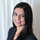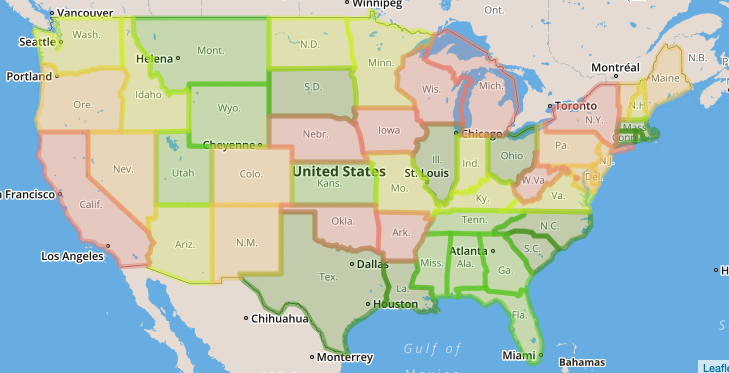# Visualizing Densities on a MapWith maps, I like to use color to easily show relative densities and concentration. For this, use a case when statement to split your regions into groups, like so:

```case
when density <= 10
then '1-10'
when density <= 20
then '11-20'
when density <= 30
then '21-30'
when density <= 40
then '31-40'
else '41+'
end as density_group```

Or, you can split out your groups by percentile rather than by absolute value. Here's a parameterized snippet called heatmap_labels(value_field) that you can use to accomplish that

```case when [value_field] <= PERCENTILE_DISC(0.1) WITHIN GROUP (ORDER BY [value_field]) OVER () then '10%'
when [value_field] <= PERCENTILE_DISC(0.2) WITHIN GROUP (ORDER BY [value_field]) OVER () then '20%'
when [value_field] <= PERCENTILE_DISC(0.3) WITHIN GROUP (ORDER BY [value_field]) OVER () then '30%'
when [value_field] <= PERCENTILE_DISC(0.4) WITHIN GROUP (ORDER BY [value_field]) OVER () then '40%'
when [value_field] <= PERCENTILE_DISC(0.5) WITHIN GROUP (ORDER BY [value_field]) OVER () then '50%'
when [value_field] <= PERCENTILE_DISC(0.6) WITHIN GROUP (ORDER BY [value_field]) OVER () then '60%'
when [value_field] <= PERCENTILE_DISC(0.7) WITHIN GROUP (ORDER BY [value_field]) OVER () then '70%'
when [value_field] <= PERCENTILE_DISC(0.8) WITHIN GROUP (ORDER BY [value_field]) OVER () then '80%'
when [value_field] <= PERCENTILE_DISC(0.9) WITHIN GROUP (ORDER BY [value_field]) OVER () then '90%'
when [value_field] <= PERCENTILE_DISC(1) WITHIN GROUP (ORDER BY [value_field]) OVER () then '100%'
else null end as heatmap_labels```

Then, assign color values that intuitively communicate relative densities, blue/green being low-density and red being high density.

This approach works for both GeoJSON and lat/long data.